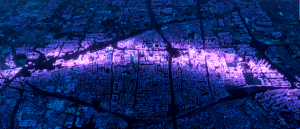# 使用three.js实现炫酷的3D城市扫光效果varying vec4 v_position;
#include <common>
#include <logdepthbuf_pars_vertex>//这个是为了支持logDepth
#ifdef USE_RELATIVE
uniform mat4 relativeModelMatrix;
#endif

void main() {

vec4 pos = vec4(position, 1.0);

gl_Position = projectionMatrix * modelViewMatrix * pos;
#include <logdepthbuf_vertex>

#ifdef USE_RELATIVE
v_position = relativeModelMatrix * pos;
#else
v_position = pos;
#endif

}

uniform vec3 color;
uniform vec3 maxPos;
uniform vec3 minPos;
varying vec4 v_position;
#ifdef USE_UVMAP
uniform sampler2D uvMap;
#endif
uniform vec3 direction;
#include <logdepthbuf_pars_fragment>
bool isMax(vec3 a, vec3 b){
float ax = a.x;
float ay = a.y;
float az = a.z;
float bx = b.x;
float by = b.y;
float bz = b.z;
return ax > bx && ay > by && az > bz;
}
bool isMin(vec3 a, vec3 b){
float ax = a.x;
float ay = a.y;
float az = a.z;
float bx = b.x;
float by = b.y;
float bz = b.z;
return ax < bx && ay < by && az < bz;
}
void main() {
#include <logdepthbuf_fragment>
float subb = length(maxPos.z – minPos.z);
float gap = length(v_position.z – minPos.z);
vec2 texCoord = vec2((gap / subb), 0.5);
gl_FragColor = mix(vec4(0,0,0,0),vec4(color, 1.0),float(isMin(v_position.xyz,maxPos) && isMax(v_position.xyz,minPos)));
#ifdef USE_UVMAP
gl_FragColor.a *= texture2D( uvMap, texCoord ).r;//可以传入一个渐变贴图，防止扫光太生硬
#endif
}

2.性能也不错
3.通用性强，任意的物体你都可以扫

2.如果要实现圆形扫光或者任意图形扫光又要再写一个材质，用户很难自定义。#include <common>
#include <logdepthbuf_pars_vertex>
varying vec2 vp;
uniform mat3 uvTransform;
attribute vec2 coordinates;
void main() {
vec4 pos = vec4(position, 1.0);
vec2 vUv = ( uvTransform * vec3( coordinates, 1 ) ).xy;
vp = vUv;
gl_Position = projectionMatrix * modelViewMatrix * pos;
#include <logdepthbuf_vertex>
}

varying vec2 vp;
uniform vec3 scanningColor;
uniform sampler2D map;

#include <logdepthbuf_pars_fragment>

void main(){
#include <logdepthbuf_fragment>
float r = texture2D(map, vp).r;
gl_FragColor = r * vec4(scanningColor, 1.0);
}————————————————

WEBGL学习网（WebGLStudy.COM）专注提供WebGL 、ThreeJS、BabylonJS等WEB3D开发案例源码下载。

1. 本站部分资源来源于用户上传和网络，如有侵权请邮件联系站长：1218436398@qq.com！我们将尽快处理。
2. 分享目的仅供大家学习和交流，您必须在下载后24小时内删除！
3. 不得使用于非法商业用途，不得违反国家法律。否则后果自负！
4. 本站提供的源码、模板、插件等等其他资源，都不包含技术服务请大家谅解！
5. 如有链接无法下载、失效或广告，请联系管理员处理！
6. 本站资源打赏售价用于赞助本站提供的服务支出（包括不限服务器、网络带宽等费用支出）！
7.欢迎加QQ群学习交流：549297468 ，或者搜索微信公众号：WebGL学习网
WEBGL学习网 » 使用three.js实现炫酷的3D城市扫光效果